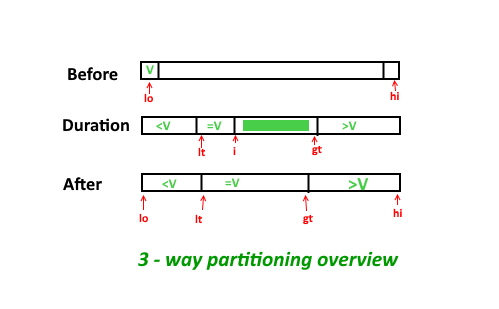Related Articles

# Three way partitioning of an array around a given range

• Difficulty Level : Medium
• Last Updated : 18 May, 2021

Given an array and a range [lowVal, highVal], partition the array around the range such that array is divided in three parts.
1) All elements smaller than lowVal come first.
2) All elements in range lowVal to highVVal come next.
3) All elements greater than highVVal appear in the end.
The individual elements of three sets can appear in any order.

Examples:

```Input: arr[] = {1, 14, 5, 20, 4, 2, 54, 20, 87, 98, 3, 1, 32}
lowVal = 14, highVal = 20
Output: arr[] = {1, 5, 4, 2, 1, 3, 14, 20, 20, 98, 87, 32, 54}

Input: arr[] = {1, 14, 5, 20, 4, 2, 54, 20, 87, 98, 3, 1, 32}
lowVal = 20, highVal = 20
Output: arr[] = {1, 14, 5, 4, 2, 1, 3, 20, 20, 98, 87, 32, 54} ```A simple solution is to sort the array. This solution does a lot of extra rearrangements and requires O(n Log n) time.
An efficient solution is based on Dutch National Flag based QuickSort. We traverse given array elements from left. We keep track of two pointers, first (called start in below code) to store next position of smaller element (smaller than range) from beginning; and second (called end in below code) to store next position of greater element from end.

## C++

 `// C++ program to implement three way partitioning``// around a given range.``#include``using` `namespace` `std;` `// Partitions arr[0..n-1] around [lowVal..highVal]``void` `threeWayPartition(``int` `arr[], ``int` `n,``                ``int` `lowVal, ``int` `highVal)``{``    ``// Initialize ext available positions for``    ``// smaller (than range) and greater lements``    ``int` `start = 0, end = n-1;` `    ``// Traverse elements from left``    ``for` `(``int` `i=0; i<=end;)``    ``{``        ``// If current element is smaller than``        ``// range, put it on next available smaller``        ``// position.``        ``if` `(arr[i] < lowVal)``            ``swap(arr[i++], arr[start++]);` `        ``// If current element is greater than``        ``// range, put it on next available greater``        ``// position.``        ``else` `if` `(arr[i] > highVal)``            ``swap(arr[i], arr[end--]);` `        ``else``            ``i++;``    ``}``}` `// Driver code``int` `main()``{``    ``int` `arr[] = {1, 14, 5, 20, 4, 2, 54, 20, 87,``                ``98, 3, 1, 32};``    ``int` `n = ``sizeof``(arr)/``sizeof``(arr);` `    ``threeWayPartition(arr, n, 10, 20);` `    ``cout << ``"Modified array \n"``;``    ``for` `(``int` `i=0; i

## Java

 `// Java program to implement three way partitioning``// around a given range.``import` `java.io.*;` `class` `GFG``{``    ` `    ``// Partitions arr[0..n-1] around [lowVal..highVal]``    ``public` `static` `void` `threeWayPartition(``int``[] arr, ``int` `lowVal, ``int` `highVal)``    ``{``        ` `        ``int`  `n = arr.length;``        ` `        ``// Initialize ext available positions for``        ``// smaller (than range) and greater lements``        ``int` `start = ``0``, end = n-``1``;``        ` `         ``// Traverse elements from left``        ``for``(``int` `i = ``0``; i <= end;)``        ``{``            ` `            ``// If current element is smaller than``            ``// range, put it on next available smaller``            ``// position.``            ` `            ``if``(arr[i] < lowVal)``            ``{``                ` `                ``int` `temp = arr[start];``                ``arr[start] = arr[i];``                ``arr[i] = temp;``                ``start++;``                ``i++;``                ` `            ``}``            ` `            ``// If current element is greater than``            ``// range, put it on next available greater``            ``// position.``            ``else` `if``(arr[i] > highVal)``            ``{``                ` `                ``int` `temp = arr[end];``                ``arr[end] = arr[i];``                ``arr[i] = temp;``                ``end--;``                 ` `            ``}``            ` `            ``else``               ``i++;``        ``}``        ` `    ``}``    ` `    ``public` `static` `void` `main (String[] args)``    ``{``    ` `    ` `        ``int` `arr[] = {``1``, ``14``, ``5``, ``20``, ``4``, ``2``, ``54``, ``20``, ``87``, ``98``, ``3``, ``1``, ``32``};``        ` `        ``threeWayPartition(arr, ``10``, ``20``);`` ` `        ``System.out.println(``"Modified array "``);``        ``for` `(``int` `i=``0``; i < arr.length; i++)``        ``{``            ``System.out.print(arr[i] + ``" "``);``    ` `        ``}   ``    ``}``}` `//This code is contributed by Dheerendra Singh`

## Python3

 `# Python3 program to implement three way``# partitioning around a given range.` `# Partitions arr[0..n-1] around [lowVal..highVal]``def` `threeWayPartition(arr, n, lowVal, highVal):` `    ``# Initialize ext available positions for``    ``# smaller (than range) and greater lements``    ``start ``=` `0``    ``end ``=` `n ``-` `1``    ``i ``=` `0` `    ``# Traverse elements from left``    ``while` `i <``=` `end:` `        ``# If current element is smaller than``        ``# range, put it on next available smaller``        ``# position.``        ``if` `arr[i] < lowVal:``            ``temp ``=` `arr[i]``            ``arr[i] ``=` `arr[start]``            ``arr[start] ``=` `temp``            ``i ``+``=` `1``            ``start ``+``=` `1` `        ``# If current element is greater than``        ``# range, put it on next available greater``        ``# position.``        ``elif` `arr[i] > highVal:``            ``temp ``=` `arr[i]``            ``arr[i] ``=` `arr[end]``            ``arr[end] ``=` `temp``            ``end ``-``=` `1` `        ``else``:``            ``i ``+``=` `1` `# Driver code``if` `__name__ ``=``=` `"__main__"``:``    ``arr ``=` `[``1``, ``14``, ``5``, ``20``, ``4``, ``2``, ``54``,``           ``20``, ``87``, ``98``, ``3``, ``1``, ``32``]``    ``n ``=` `len``(arr)` `    ``threeWayPartition(arr, n, ``10``, ``20``)` `    ``print``(``"Modified array"``)``    ``for` `i ``in` `range``(n):``        ``print``(arr[i], end ``=` `" "``)` `# This code is contributed by``# sanjeev2552`

## C#

 `// C# program to implement three way``// partitioning around a given range.``using` `System;` `class` `GFG {` `    ``// Partitions arr[0..n-1]``    ``// around [lowVal..highVal]``    ``public` `static` `void` `threeWayPartition(``int``[] arr,``                           ``int` `lowVal, ``int` `highVal)``    ``{``        ``int` `n = arr.Length;` `        ``// Initialize ext available positions for``        ``// smaller (than range) and greater lements``        ``int` `start = 0, end = n - 1;` `        ``// Traverse elements from left``        ``for` `(``int` `i = 0; i <= end;) {` `            ``// If current element is smaller than``            ``// range, put it on next available smaller``            ``// position.` `            ``if` `(arr[i] < lowVal) {` `                ``int` `temp = arr[start];``                ``arr[start] = arr[i];``                ``arr[i] = temp;``                ``start++;``                ``i++;``            ``}` `            ``// If current element is greater than``            ``// range, put it on next available greater``            ``// position.``            ``else` `if` `(arr[i] > highVal) {` `                ``int` `temp = arr[end];``                ``arr[end] = arr[i];``                ``arr[i] = temp;``                ``end--;``            ``}` `            ``else``                ``i++;``        ``}``    ``}``    ` `    ``// Driver code``    ``public` `static` `void` `Main()``    ``{``        ``int``[] arr = { 1, 14, 5, 20, 4, 2, 54,``                      ``20, 87, 98, 3, 1, 32 };` `        ``threeWayPartition(arr, 10, 20);` `        ``Console.WriteLine(``"Modified array "``);``        ``for` `(``int` `i = 0; i < arr.Length; i++) {``            ``Console.Write(arr[i] + ``" "``);``        ``}``    ``}``}` `// This article is contributed by vt_m.`

## Javascript

 ``

Output:

```Modified array
1 5 4 2 1 3 14 20 20 98 87 32 54 ```

Time Complexity: O(n)
Auxiliary Space: O(1)